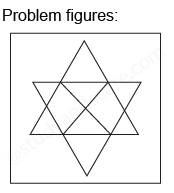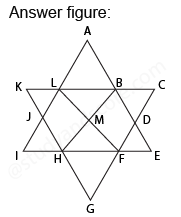## Practice Test Discussion

Q)

In each of the following question, count the number of parallelogram in the given figure.• ExplanationAfter labelling the figure, we get the following,

• Simplest parallelograms LMHJ and BDFM (2)
• Parallelograms with two components each ABML and MFGH (2)
• Prallelograms with three components each LBHI, LBEF, BDGH, DFLA, BCFH, KLFH, ABHJ and LFGJ (8)
• Parallelogram composed of six components is LCFI, KBEH and ADGJ (3)

Total number of parallelograms in the figure is 2 + 2 + 8 + 3 = 15

Hence option D is correct.

- Share with your friends! -